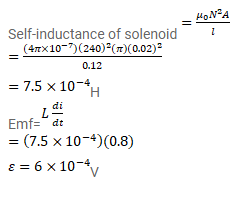# The current in a solenoid of 240 turns,

Question:

The current in a solenoid of 240 turns, having a length of $12 \mathrm{~cm}$ and a radius of $2 \mathrm{~cm}$, changes at a rate of $0.8 \mathrm{~A} / \mathrm{s}$. Find the emf induced in it.

Solution: